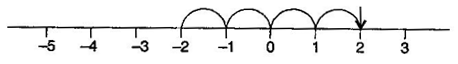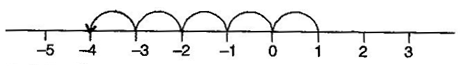### Integers-Solutions Ex-6.1

CBSE Class –VI Mathematics
NCERT Solutions
Chapter 6
Integers (Ex. 6.1)

Question 1. Write opposite of the following:
(a) Increase in weight
(b) 30 km north
(c) 326 BC
(d) Loss of Rs. 700
(e) 100 m above sea level
(b) 30 km south
(d) Profit of Rs. 700
(e) 100 m below sea level
Question 2. Represent the following numbers as integers with appropriate signs.
(a) An aeroplane is flying at a height two thousand meters above the ground.
(b) A submarine is moving at a depth eight thousand meters below the sea level.
(c) A deposit of rupees two hundred.
(d) Withdrawal of rupees seven hundred.
(b) (–) 800 meters
(c) (+) 200 Rupees
(d) (–) 700 Rupees
Question 3. Represent the following numbers on number line:
(a) +5
(b) –10
(c) +8
(d) –1
(e) –6
(a)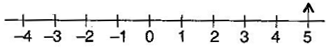(b)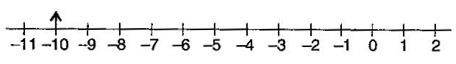(c)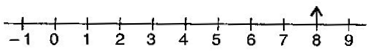(d)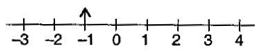(e)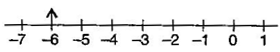Question 4. Adjacent figure is a vertical number line, representing integers. Observe it and locate the following points: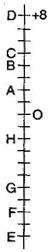(a) If point D is +8 then which point is –8?
(b) Is point G a negative integer or a positive integer?
(c) Write integers for points B and E.
(d) Which point marked on this number line has the least value?
(e) Arrange all the points in decreasing order of values.
(b) Negative
(c) B = (+) 4; E = (–) 10
(d) E
(e) D, C, B, A, O, H, G, F, E
Question 5. Following is the list of temperatures of five places in India, on a particular day of the year.
 Place Temperature Siachin 10∘${}^{\circ }$ C below 0∘${}^{\circ }$C _____________ Shimla 2∘${}^{\circ }$C below 0∘${}^{\circ }$C _____________ Ahmedabad 30∘${}^{\circ }$C above 0∘${}^{\circ }$C _____________ Delhi 20∘${}^{\circ }$C above 0∘${}^{\circ }$C _____________ Srinagar 5∘${}^{\circ }$C below 0∘${}^{\circ }$C _____________
(a) Write the temperature of these places in the form of integers in the blank column.
(b) Following is the number line representing the temperature in degree Celsius.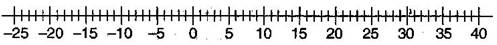Plot the name of the city against its temperature.
(c) Which is the coolest place?
(d) Write the names of the places where the temperature are above 10oC.
 Place Temperature Siachin (-) 10∘${}^{\circ }$C Shimla (–) 2∘${}^{\circ }$C Ahmedabad (+) 30∘${}^{\circ }$C Delhi (+) 20∘${}^{\circ }$C Srinagar (–) 5∘${}^{\circ }$C
(b) Number line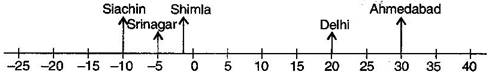(c) Siachin
Question 6. In each of the following pairs, which number is to the right of the other on the number line?
(a) 2, 9
(b) –3, –8
(c) 0, –1
(d) –11, 10
(e) –6, 6
(f) 1, –100
Answer: (a) 9 is right to 2
(b) –3 is right to –8
(c) 0 is right to –1
(d) 10 is right to –11
(e) 6 is right to –6
(f) 1 is right to –100
Question 7. Write all the integers between the given pairs (write them in the increasing order):
(a) 0 and –7(b) –4 and 4
(c) –8 and –15
(d) –30 and –23
Answer: (a) –6, –5, –4, –3, –2, –1
(b) –3, –2, –1, 0, 1, 2, 3
(c) –14, –13, –12, –11, –10, –9
(d) –29, –28, –27, –26, –25, –24
Question 8. (a) Write four negative integers greater than –20.
(b) Write four negative integers less than –10.
Answer: (a) –19, –18, –17, –16
(b) –11, –12, –13, –14
Question 9. For the following statements write True (T) or False (F). If the statement is false, correct the statement:
(a) –8 is to the right of –10 on a number line.
(b) –100 is the right of –50 on a number line.
(c) Smallest negative integer is –1.
(d) –26 is larger than –25.
(b) False [ –50 is the right of –100 on a number line.]
(c) False [ Smallest negative integer is – infinity]
(d) False [–26 is smaller than –25.]
Question 10. Draw a number line and answer the following:
(a) Which number will we reach if we move 4 numbers to the right of –2?
(b) Which number will we reach if we move 5 numbers to the left of 1?
(c) If we are at –8 on the number line, in which direction should we move to reach –13?
(d) If we are at –6 on the number line, in which direction should we move to reach –1?